# Python | Pandas dataframe.reindex_like()

• Last Updated : 22 Nov, 2018

Python is a great language for doing data analysis, primarily because of the fantastic ecosystem of data-centric python packages. Pandas is one of those packages and makes importing and analyzing data much easier.

Pandas` dataframe.reindex_like()` function return an object with matching indices to myself. Any non-matching indexes are filled with `NaN` values.

Attention geek! Strengthen your foundations with the Python Programming Foundation Course and learn the basics.

To begin with, your interview preparations Enhance your Data Structures concepts with the Python DS Course. And to begin with your Machine Learning Journey, join the Machine Learning - Basic Level Course

Syntax:
Syntax : DataFrame.reindex_like(other, method=None, copy=True, limit=None, tolerance=None)

Parameters :
other : Object
method : string or None
copy : boolean, default True
limit : Maximum number of consecutive labels to fill for inexact matches.
tolerance : Maximum distance between labels of the other object and this object for inexact matches. Can be list-like.

Returns : reindexed : same as input

Example #1: Use `reindex_like()` function to find the matching indexes between the given two dataframes.

Note : We can fill in the missing values by using any of the fill methods (ex. ‘ffill’, ‘bfill’).

 `# importing pandas as pd``import` `pandas as pd`` ` `# Creating the first dataframe ``df1 ``=` `pd.DataFrame({``"A"``:[``1``, ``5``, ``3``, ``4``, ``2``],``                    ``"B"``:[``3``, ``2``, ``4``, ``3``, ``4``],``                    ``"C"``:[``2``, ``2``, ``7``, ``3``, ``4``],``                    ``"D"``:[``4``, ``3``, ``6``, ``12``, ``7``]},``                    ``index ``=``[``"A1"``, ``"A2"``, ``"A3"``, ``"A4"``, ``"A5"``])`` ` `# Creating the second dataframe``df2 ``=` `pd.DataFrame({``"A"``:[``10``, ``11``, ``7``, ``8``, ``5``], ``                    ``"B"``:[``21``, ``5``, ``32``, ``4``, ``6``],``                    ``"C"``:[``11``, ``21``, ``23``, ``7``, ``9``], ``                    ``"D"``:[``1``, ``5``, ``3``, ``8``, ``6``]}, ``                     ``index ``=``[``"A1"``, ``"A3"``, ``"A4"``, ``"A7"``, ``"A8"``])`` ` `# Print the first dataframe``df1`` ` `# Print the second dataframe``df2`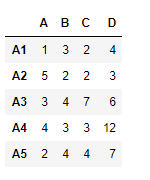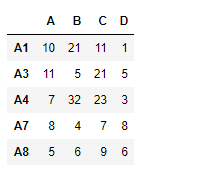Let’s use the `dataframe.reindex_like()` function to find the matching indexes.

 `# find matching indexes``df1.reindex_like(df2)`

Output :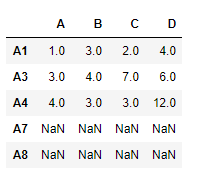Notice the output, unmatched indexes are populated with `NaN` values, we can fill in the missing values using ‘ffill’ method.

 `# filling the missing values using ffill method``df1.reindex_like(df2, method ``=``'ffill'``)`

Output :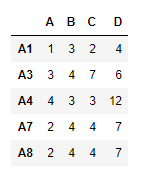Notice in the output, the new indexes has been populated using the “A5” row.

Example #2: Use `reindex_like()` function to match the indexes of two dataframes with limit on filling the missing values.

 `# importing pandas as pd``import` `pandas as pd`` ` `# Creating the first dataframe ``df1 ``=` `pd.DataFrame({``"A"``:[``1``, ``5``, ``3``, ``4``, ``2``],``                    ``"B"``:[``3``, ``2``, ``4``, ``3``, ``4``],``                    ``"C"``:[``2``, ``2``, ``7``, ``3``, ``4``],``                    ``"D"``:[``4``, ``3``, ``6``, ``12``, ``7``]},``                    ``index ``=``[``"A1"``, ``"A2"``, ``"A3"``, ``"A4"``, ``"A5"``])`` ` `# Creating the second dataframe``df2 ``=` `pd.DataFrame({``"A"``:[``10``, ``11``, ``7``, ``8``, ``5``],``                    ``"B"``:[``21``, ``5``, ``32``, ``4``, ``6``],``                    ``"F"``:[``11``, ``21``, ``23``, ``7``, ``9``],``                    ``"K"``:[``1``, ``5``, ``3``, ``8``, ``6``]},``                    ``index ``=``[``"A1"``, ``"A2"``, ``"A3"``, ``"A4"``, ``"A7"``])`` ` `# matching the indexes``df1.reindex_like(df2)`

Output :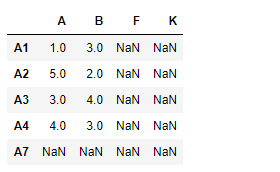Notice the output, unmatched indexes are populated with `NaN` values, we can fill in the missing values using ‘ffill’ method. we also limit the number of consecutive unmatched indexes that could be filled using the limit parameter.

 `# match the indexes``# fill the unmatched index using 'ffill' method``# maximum consecutive unmatched indexes to be filled is 1`` ` `df.reindex_like(df1, method ``=``'ffill'``, limit ``=` `1``)`

Output :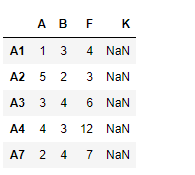My Personal Notes arrow_drop_up